Brain teasers / Math Puzzles / with Numbers

# Mathematical Number Puzzles

Number Puzzles help children learn to add and subtract, arrange numbers in ascending and descending order.

## Number Puzzles to train math skills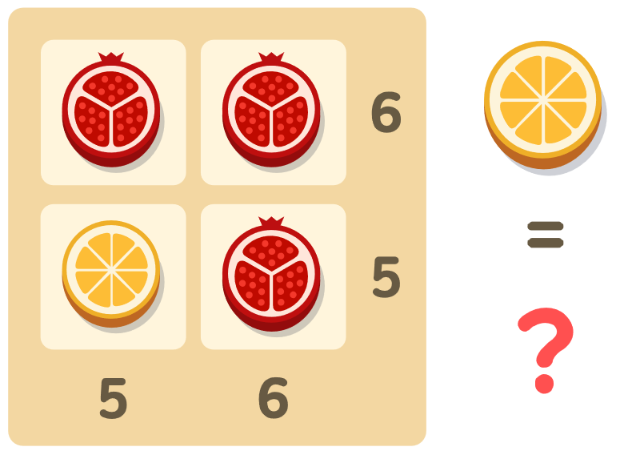Mathematical rebus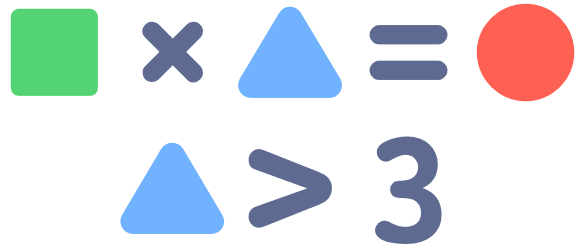Which digits can a square be equal to?
1, 2, 3 or 4?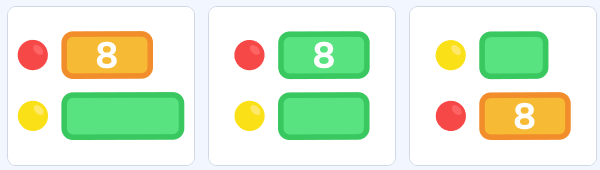There are 8 red balls, and there are more yellow balls than red ones. Choose the appropriate scheme.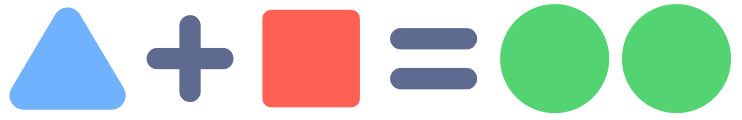What numbers are a triangle and a square equal to?
Answer options: Both even / Both odd / One even and one odd.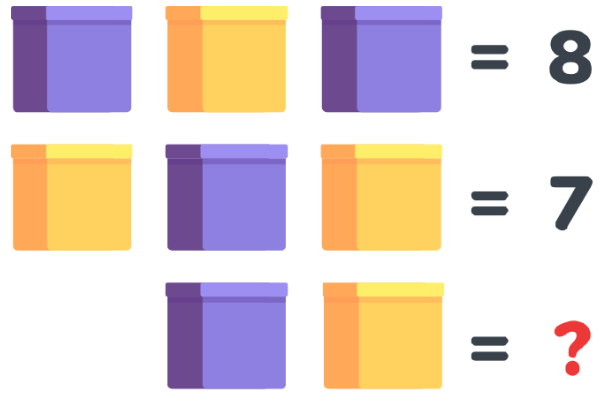Math rebus